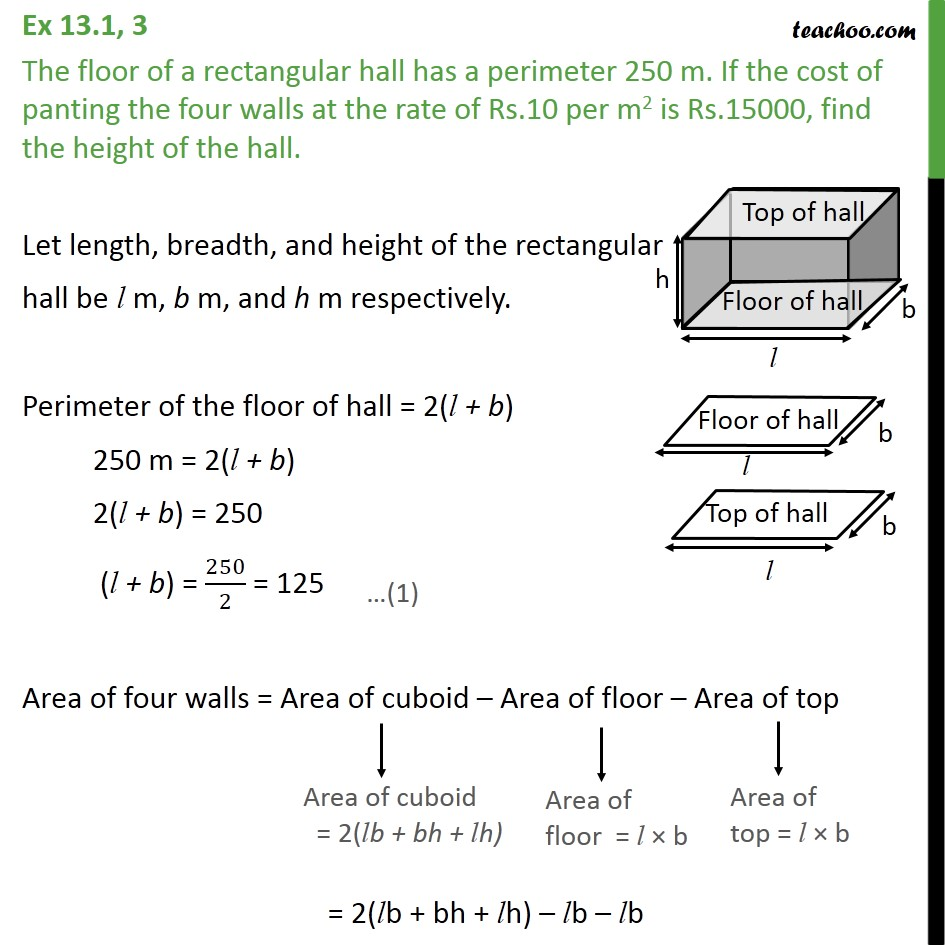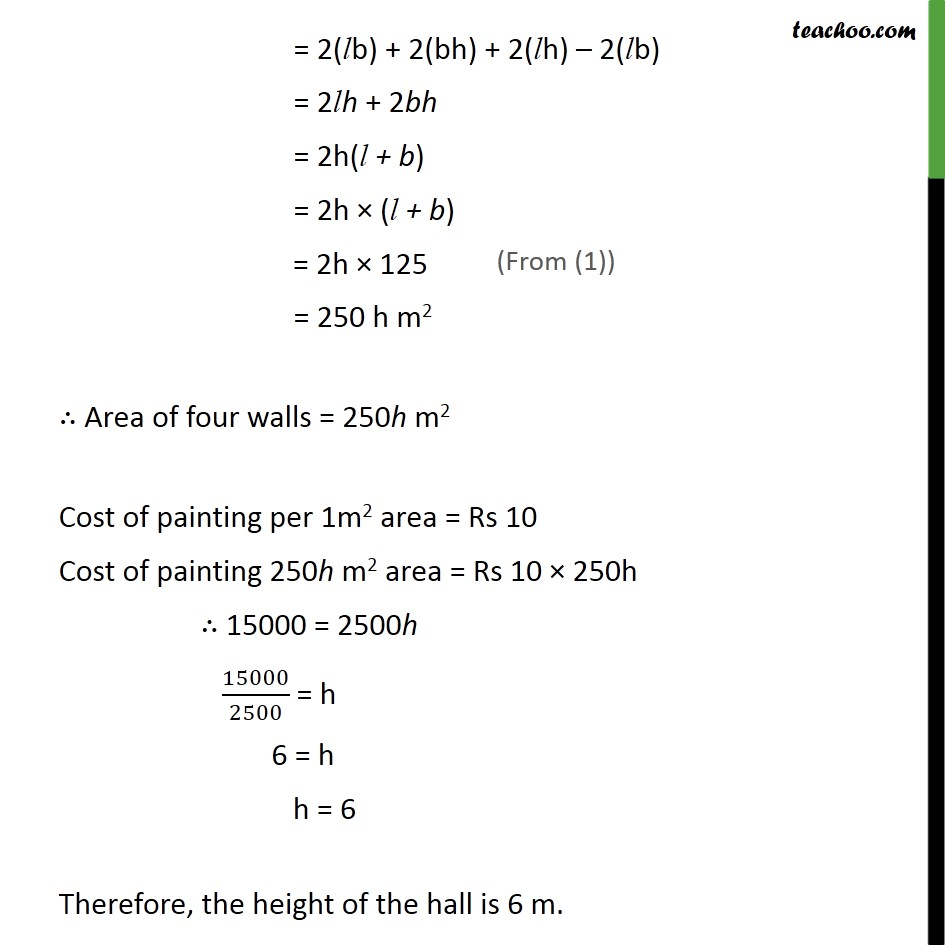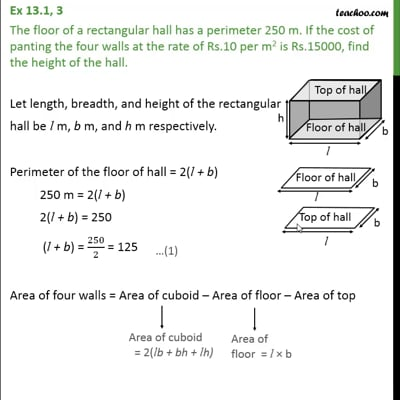Ex 13.1

Chapter 13 Class 9 Surface Areas and Volumes (Term 2)
Serial order wiseThis video is only available for Teachoo black users

### Transcript

Ex 13.1, 3 The floor of a rectangular hall has a perimeter 250 m. If the cost of panting the four walls at the rate of Rs.10 per m2 is Rs.15000, find the height of the hall. Let length, breadth, and height of the rectangular hall be l m, b m, and h m respectively. Perimeter of the floor of hall = 2(l + b) 250 m = 2(l + b) 2(l + b) = 250 (l + b) = 250/2 = 125 Area of four walls = Area of cuboid – Area of floor – Area of top = 2(lb + bh + lh) – lb – lb = 2(lb) + 2(bh) + 2(lh) – 2(lb) = 2lh + 2bh = 2h(l + b) = 2h × (l + b) = 2h × 125 = 250 h m2 ∴ Area of four walls = 250h m2 Cost of painting per 1m2 area = Rs 10 Cost of painting 250h m2 area = Rs 10 × 250h ∴ 15000 = 2500h 15000/2500 = h 6 = h h = 6 Therefore, the height of the hall is 6 m.